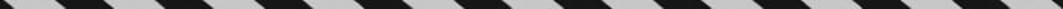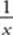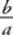﻿ ﻿ARITHMETIC OPERATIONS WITH FRACTIONS - Fractions, Decimals, and Percents - TOPICS IN ARITHMETIC - SAT SUBJECT TEST MATH LEVEL 1

## TOPICS IN ARITHMETIC## CHAPTER 3Fractions, Decimals, and Percents### ARITHMETIC OPERATIONS WITH FRACTIONS

Key Fact B4

To multiply two fractions, multiply their numerators and multiply their denominators:Key Fact B5

To multiply a fraction by any other number, write that number as a fraction whose denominator is 1:TIPAll calculations with fractions should be done on your calculator. So you really don”t need to use these rules with numbers. However, on the Math 1 test, you might have to multiply algebraic fractions such as, which you cannot do on your calculator.

 TACTICB1 Before multiplying fractions, reduce. You may reduce by dividing any numerator and any denominator by a common factor.

EXAMPLE 5:, and as you will see in Chapter 5:TACTICB2 When a problem requires you to find a fraction of a number, multiply. Since a percent is just a fraction whose denominator is 100, you also multiply to find a percent of a number.

EXAMPLE 6: Ifof the 840 students at Monroe High School are freshmen and if 30% of the freshmen play musical instruments, how many freshmen play musical instruments?There arefreshmen. Of these, 30% play an instrument:The reciprocal of any nonzero number, x, is the number. The reciprocal of the fractionis the fraction.

Key Fact B6

To divide any number by a fraction, multiply that number by the reciprocal of the fraction.Key Fact B7

To add or subtract fractions with the same denominator, add or subtract the numerators and keep the denominator.Key Fact B8

To add or subtract fractions with different denominators, first rewrite the fractions as equivalent fractions with the same denominators.NOTE: The easiest denominator to get is the product of the denominators (64 = 24, in the example). However, the best denominator to use is the least common denominator, which is the least common multiple (LCM) of the denominators (12 in this case).

Smart Strategy

Using the least common denominator minimizes the amount of reducing necessary to express an answer in lowest terms.

Key Fact B9

Ifis the fraction of the whole that satisfies some property, then 1–is the fraction of the whole that does not satisfy it.

EXAMPLE 7: In a jar,of the marbles are red,are white, andare blue. What fraction of the marbles are neither red, white, nor blue?

The red, white, and blue marbles constituteof the total. So,1 of the marbles are neither red, white, nor blue.

EXAMPLE 8: There are 800 students at Central High School, each of whom takes exactly one of four science courses. Iftake earth science,take biology, andtake chemistry, how many students take physics?

Sinceof the students take a science other than physics andtake physics. Finally,students take physics.

﻿